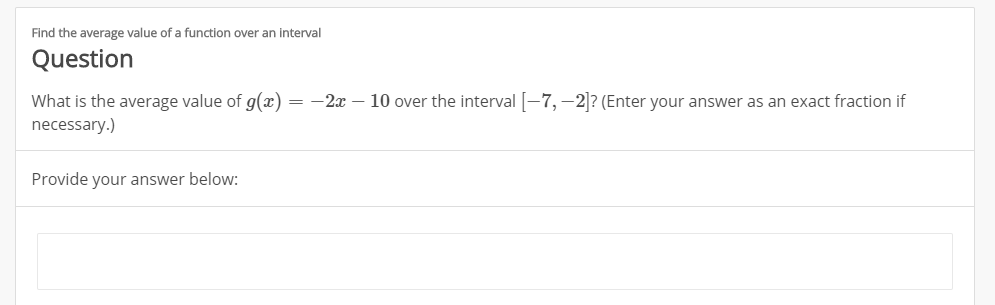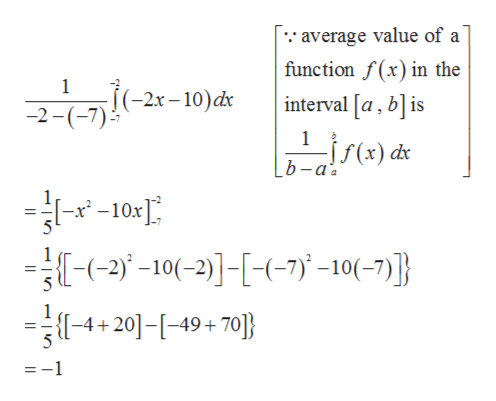Find the average value of a function over an intervalQuestionWhat is the average value of g(x) = -2x - 10 over the interval-7,-2? (Enter your answer as an exact fraction ifnecessary.)Provide your answer below:

Questionhelp_outlineImage TranscriptioncloseFind the average value of a function over an interval Question What is the average value of g(x) = -2x - 10 over the interval-7,-2? (Enter your answer as an exact fraction if necessary.) Provide your answer below: fullscreen

Average value of the gi...help_outlineImage Transcriptioncloseaverage value of a function f(x) in the 1 (-2x-10)dx interval [a, bis -2 1 f(x) dx b -a 10: 2-10(-2)7-10(-7)} -4+20]-[-49+70} :-1 fullscreen

Want to see the full answer?

See Solution

Want to see this answer and more?

Our solutions are written by experts, many with advanced degrees, and available 24/7

See Solution
Tagged in

Calculus